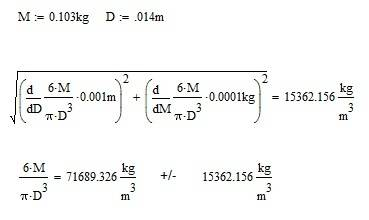# Finding Uncertainties

Paymemoney

## Homework Statement

Calculate the density p and its uncertainty $$\Delta$$p showing all working

Mass M (kg)
0.0163

$$\Delta{M}$$ (kg)
?

Diameter D (m)
0.014

$$\Delta{D}$$ (m)
?

## Homework Equations

$$\rho=MV$$
$$p= \frac{6M}{\Pi{D}^3}$$
$$Z=\frac{A^2B}{3C^{0.5}}$$
$$\frac{\Delta{Z}}{Z}=\frac{2\Delta{A}}{A}+\frac{\Delta{B}}{B}+(1/2)\frac{\Delta{C}}{C}$$

## The Attempt at a Solution

I have tried to use $$p= \frac{6M}{\Pi{D}^3}$$ but i don't think this is the equation to get the answer.
To find $$\Delta{p}$$, I don't understand how i can find $$\Delta{p}$$ if i do not have $$\Delta{m}$$ and $$\Delta{D}$$(which i don't understand how to get) from the equation:

$$\frac{\Delta{p}}{p}=\frac{\Delta{m}}{m}+\frac{\Delta{D}}{D}$$

Hope someone can help me
P.S Paymemoney

Harry Hazard
You are indeed in a pickle. I've never seen an uncertainty analysis like this.

Do you know the resolution of the measuring devices? The resolution is the "smallest marking
" on your measuring device. For example: Most rulers have 1/16th of an inch as the smallest mark, therefore the resolution would be 1/16th of an inch.

Once you know that, you should be able to preform a uncertainty analysis using the Kline and McClintock method.

An example can be found http://lyle.smu.edu/me/2142/uncert/uncert.htm"

I feel like this will not answer your question because I've never seen a uncertainty method like this.

Taking a compete stab in the dark, and making quite a few assumptions.

I assume that since kg is measured to .0103, that these are all significant digits and that the resolution is .0001 kg. Therefore, the zeroth-order uncertainty is .0001/2 kg = .00005kg.

Similarly for the diameter. .014, all significant. The uncertainty1 is .001 m. The Zeroth-Order uncertainty is .001m/2=.0005m

See AttachmentLast edited by a moderator: